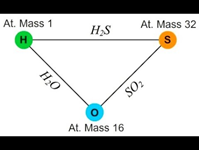# Laws of StoichiometryLaws of stoichiometry

·         The laws which deal with the composition of substance by mass or by volume are called the laws of stoichiometry.

·         The compounds in which atoms are combined in exact whole number ratio are known as stoichiometric compounds.

The laws of stoichiometry are:

1.       Law of conservation of mass

2.       Law of definite/constant proportion

3.       Law of multiple proportion

4.       Law of reciprocal proportions

5.       Law of gaseous volume

1.       Law of conservation of mass

Ø  Propounded by French chemist Antoine Lavoisier in 1774

Ø  States that “mass is neither gained nor lost during a chemical reaction” or “in a chemical; reaction the total mass of reactants consumed is equal to the total mass of the products formed”

Ø  Also called law of indestructibility of matter

Ø  According to this law, if reactants A and B combine to form products C and D, then the sum of masses of A and B is equal to the sum of masses of C and D.

Ø  Limitation: Not completely applicable for nuclear reactions where mass of products formed is slightly less than the mass of the reactants due to conversion of some amount of mass into energy as per the equation E=mc2

2.       Law of definite/constant proportion

Ø  Propounded by French chemist Joseph Louis Proust in 1799

Ø  States that “the same chemical compound always contains same elements combined together in a definite proportion by mass regardless of the origin or mode of formation of the compound”

Ø  For example, carbon dioxide gas obtained either by heating of lime stone or from the combustion of carbon or hydrocarbon contains carbon and oxygen atoms combined in the ratio of 3:8 by mass regardless of their source of formation.

Ø  Limitations: 1. This law does not hold true if the element involved in the compound formation exists in different isotopes. For example, CO2 formed by the combination of C-14 isotope and oxygen contains carbon and oxygen atoms in the ratio of 7:16 by mass, unlike the first case. 2. This law is not applicable for non- stoichiometric compounds like wustite where the ratio of iron and oxygen ranges from 0.83:1 to 0.95:1.

3.       Law of multiple proportion

Ø  Propounded by John Dalton in 1803

Ø  States that “when two different elements combine to form two or more than two chemical compounds then the mass of one of the elements which combine with a fixed mass of the other bear a simple whole number ratio.”

Ø  For example, nitrogen combines with oxygen to form series of oxides of nitrogen, where the ratio of masses of oxygen that combine with 28 parts by mass of nitrogen is 1:2:3:4:5, illustrating law of multiple proportion.

 Compound Mass of nitrogen(g) Mass of oxygen(g) Mass ratio Simple whole no. ratio of mass of oxygen with constant mass of nitrogen N2O 28 16 28:16 NO 14 16 28:32 N2O3 28 48 28:48 1:2:3:4:5 N2O4 28 64 28:64 N2O5 28 80 28:80

4.       Law of reciprocal proportion

Ø  Propounded by Ritcher in 1792

Ø  States that “the ratio of the masses of two elements which combine separately with a fixed mass of the third element is either same or some simple multiple of the ratio of the masses in which these elements combine with each other”

Ø  For example, let us consider three elements hydrogen, oxygen and Sulphur. Oxygen and hydrogen combine separately with Sulphur to form SO2 and H2S. They also combine with each other to form H2O as shown in figure.

ØØ  In Sulphur dioxide(SO2), 32 parts by mass of sulphur combines with 32 parts by mass of oxygen.

Ø  In hygrogen sulphide(H2S), 2 parts by mass of hydrogen combine with 32 parts by mass of sulphur.

Ø  Therefore, the ratio of masses of hydrogen and oxygen which combine with fixed mass, i.e. 32 parts by mass of sulphur is

Ø                                                2:32 i.e. 1:16……………..(i)

Ø  In water(H2O), 2 parts by mass of hydrogen combine with 16 parts by mass of oxygen. The ratio of masses of hydrogen and oxygen is

Ø                                                 2:16 i.e. 1:8……………..(ii)

Ø  These two ratios are related as 1/16:1/8 i.e. 1:2, which is simple multiple ratio.

5.       Law of gaseous volume

Ø  Propounded by Gay Lussac in 1808, also called Gay Lussac’s law of gaseous volumes

Ø  States that “under similar conditions of temperature and pressure, when gases react together, they do so in volumes which bear a simple ratio to one another and the volumes of the products as well if they are also gases”

Ø  For example, if steam is formed by the combination of hydrogen and oxygen gas then there is a simple ratio of their volume.

Ø                        2H2(g) + O2 → 2H2O(g)

Ø  The volume ratio of reactants and products which is 2:1:2 is a simple ratio.